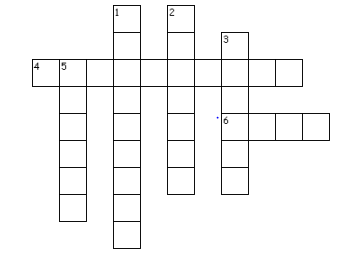# Light Worksheet

In this page we have Light worksheet for Class 7 Science . Hope you like them and do not forget to like , social share and comment at the end of the page.
Question 1
Fill the blanks:
1. The property by which light in the straight line is termed as_______________________
2. Light travels with a speed of ______________________________km/s in air.
3. The splitting of white light into its seven constituent colours is called________________
4. _____________________is the study of spectrum formed when a surface emits light.
5. _____________________was the first person who discovered that sunlight is made up of seven colours.
6. _____________________lens is present in human eye.
7. A ____________________ lens always forms an erect, virtual and diminished image.

Question 2
1. Differentiate between the following (3 points each)
a) Concave and convex mirror.
b) Real and virtual image.
1. Define a) Mirror b) lens c) prism d) rainbow e) lateral inversion
2. Which mirror is used as rear views mirror and why?
3. Why is it dangerous to look at the sun through a convex lens?
4. What is a Newton’s disc? How does it show that light is composed of many colours?
5. Why is concave mirror used in search lights & head lights?

Question 3
True and false
a. Light is the fastest travelling form of energy
b. Light travels in curve path
c. As per law of reflection, Angle of Incidence = Angle of Reflections
d. A concave lens can be used to produce an enlarged and erect image.
e. An object can be seen only if it emits light
f. A concave lens is called the converging lens

Question 4
Fill in the blanks
a. The inner surface of a spoon acts like a ________ while its outer surface acts like a _________________
b. An image formed on a screen is called a _______
c. _______mirrors used as side mirrors in scooters /cars
d. The lenses which feel thicker in the middle than at the edges are _________
e. The seven colours in rainbow are red, orange, yellow, green, blue, ______ and ________

Question 5
Multiple Choice questions
i. John and Tom were given one mirror each by their teacher. Tom found his image to be erect and of the same size whereas John found her image erect and smaller in size. This means that the mirrors of John and tom are, respectively
(a) plane mirror and concave mirror.
(b) concave mirror and convex mirror.
(c) plane mirror and convex mirror.
(d) convex mirror and plane mirror
ii. You are provided with a convex mirror, a concave mirror, a convex lens and a concave lens. You can get an inverted image rom
(a) both concave lens and convex lens.
(b) both concave mirror and convex mirror.
(c) both concave mirror and convex lens.
(d) both convex mirror and concave lens.

Question 6
Unscrambled the Jumbled word
a. EALICHPSR
b. ISRPM
c. NNOARIW
d. RVINTDEE
e. CERTE
Question 7
Crossword PuzzleAcross
4. A convex lens is _____ lens
6. An image which can be obtained on a screen
Down
1. A concave lens is _____ lens
2. An image which cannot be obtained on a screen
3. Any polished or a shining surface acts as a _______
5. One of the rainbows colours

a. Rectilinear propagation
b. 3 X 108 m/s
c. dispersion
d. Spectroscopy
e. Sir Issac Newton
f. Convex
g. Concave

a. True
b. False
c. True
d. False
e. False
f. False
a. Concave mirror and convex mirror
b. real image
c. Convex
d. Convex lens
e. indigo and violet

i. (d)
ii. (c)

a. Spherical
b. Prism
c. Rainbow
d. inverted
e. erect

1. diverging
2.virtual
3.mirror
4.converging
5.orange
6. real

• Notes
• NCERT Solutions
• Assignments